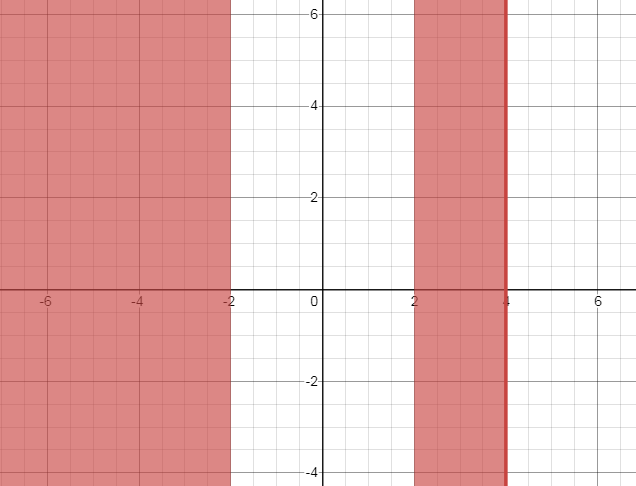# The solution of the given inequality and express the solution using interval notation. Also graph the solution set on the real number line.### Precalculus: Mathematics for Calcu...

6th Edition
Stewart + 5 others
Publisher: Cengage Learning
ISBN: 9780840068071### Precalculus: Mathematics for Calcu...

6th Edition
Stewart + 5 others
Publisher: Cengage Learning
ISBN: 9780840068071

#### Solutions

Chapter 1, Problem 91RE
To determine

## To calculate: The solution of the given inequality and express the solution using interval notation. Also graph the solution set on the real number line.

Expert Solution

In order to solve an inequality, the following things can be done.

• Add the same number to each side of the inequality.

• Subtract the same number from each side.

• Multiply or divide each side by the same number and if multiplied or divided each side by a negative number, then the inequality symbol must be reversed.

The inequality can be solved as follows:

x4x240x4(x+2)(x2)0x>2,x4,x<2

The solution using interval notation is (,2)(2,4] .

The shaded region shows solution of the given inequality on the graph below :### Explanation of Solution

Given information:

The given inequality is x4x240 .

Formula used:

In order to solve an inequality, the following things can be done.

• Add the same quantity to each side of the inequality.

• Subtract the same quantity from each side.

• Multiply or divide each side by the same positive quantity and If multiplied or divided each side by a negative quantity, then the inequality symbol must be reversed.

In case of non linear inequality,the function may be factorised and solved further long division method etc.

Calculation:

In order to solve an inequality, the following things can be done.

• Add the same quantity to each side of the inequality.

• Subtract the same quantity from each side.

• Multiply or divide each side by the same positive quantity and If multiplied or divided each side by a negative quantity, then the inequality symbol must be reversed.

The inequality can be solved as follows:

x4x240x4(x+2)(x2)0x>2,x4,x<2

The solution using interval notation is (,2)(2,4] .

The shaded region shows the given inequality on the graph below:

Calculation:

In order to solve an inequality, the following things can be done.

• Add the same quantity to each side of the inequality.

• Subtract the same quantity from each side.

• Multiply or divide each side by the same positive quantity and If multiplied or divided each side by a negative quantity, then the inequality symbol must be reversed.

The inequality can be solved as follows:

x4x240x4(x+2)(x2)0x>2,x4,x<2

The solution using interval notation is (,2)(2,4] .

The shaded region shows the given inequality on the graph below :### Have a homework question?

Subscribe to bartleby learn! Ask subject matter experts 30 homework questions each month. Plus, you’ll have access to millions of step-by-step textbook answers!